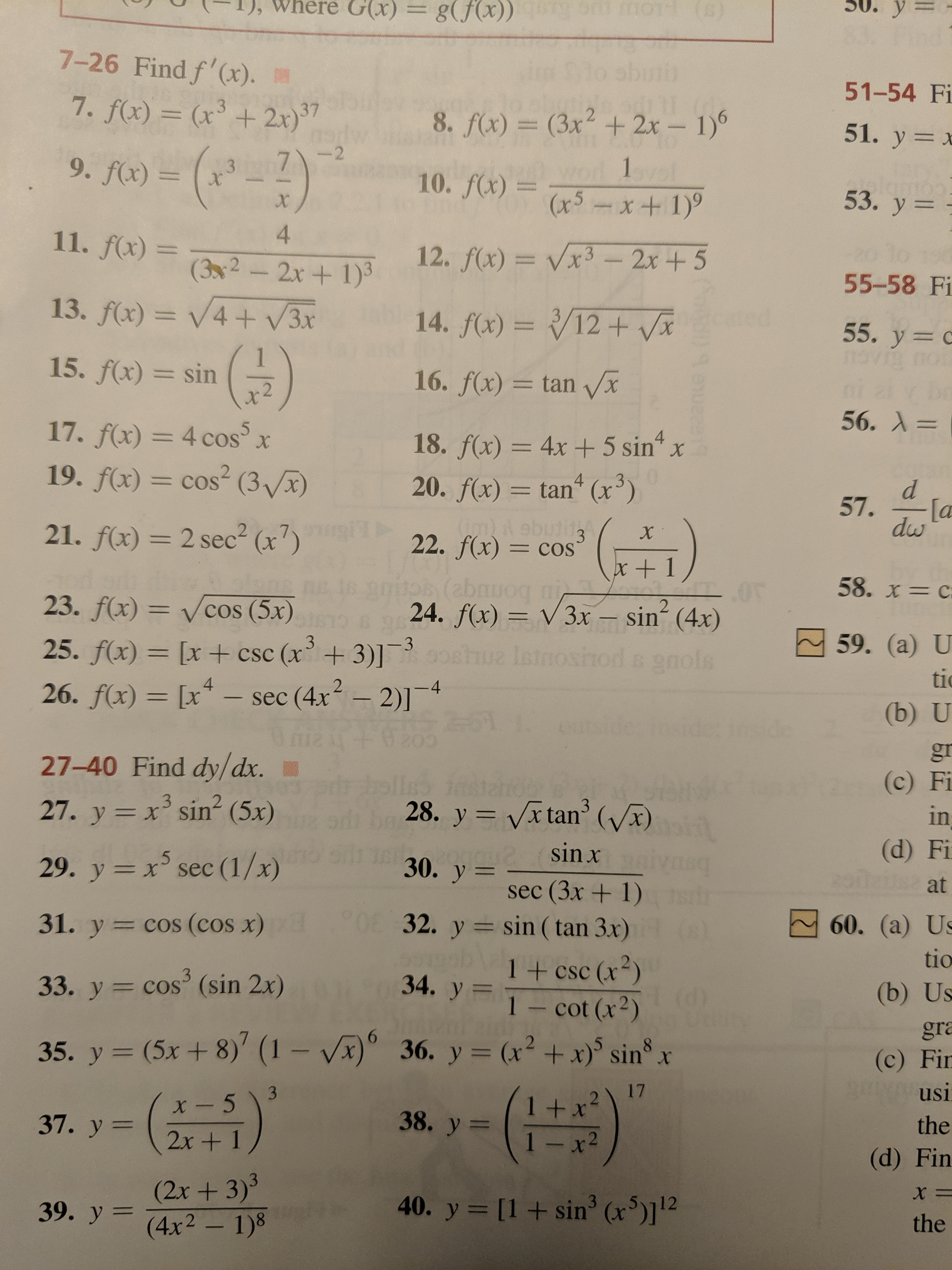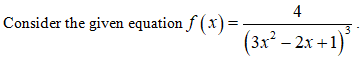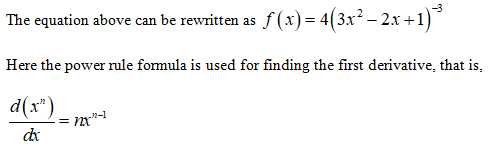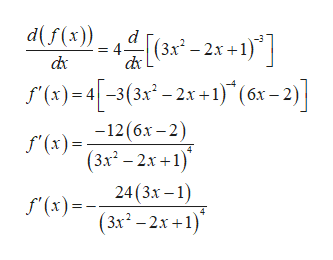# G(x)=уg(fx)) 00 o )7-26 Find f'(x).51-54 Fi7. f(x) = (x+ 2x)3728. f(x) = (3x + 2x- 1)51. y x-(-2)--279. f(x) =1pl10. f(x)x5x+ 1)3x53. у 3X411. f(x) =12. f(x)= Vx3- 2x+5(32-2x+ 1)355-58 Fi13. f(x)= V4+ V3x14. f(x)= /12+ Vx355. y covioi15. f(x) sin16. f(x) = tan Vx56. А 317. f(x) = 4 cos5X418. f(x) = 4x 5 sin20. f(x) tan (x3)19. f(x)= cos2 (3Vx)4= COSd57.[adwwOd ebutitg21. f(x) 2 sec2 (x7)X322. f(x) = cos=COSx + 158. x= C23. f(x) = Vcos (5x)24. f(x) V3x sin (4x)259. (а) U3-325. f(x)= [x+ csc (x+3)]ue Isino6 9nolstic- sec (4x2-2)12126. f(x) [x-4(b) Udegr27-40 Find dy/dx.(c) Fi327. y x sin (5x)328. y Vx tan' (x)inX(d) Fisin x530. y29. y xsec (1/x)atsec (3x+ 1)32. y sin (tan 3x)31. y cos (cos x)60. (a) Ustio1 + csc (x2)Cot (x)334. y33. y cos' (sin 2x)(b) Usgra35. y (5x + 8)' (1 - Vx)36. y= (x2 +x) sin x(c) Firusi1731 +x1 -x22Xx- 538. y37. у %3the2x+ 1(d) Fin(2x+3)339. y (4x2- 1)840. y[1sin3 (x)112theCoto

Question
15 views

#11help_outlineImage TranscriptioncloseG(x)= у g(fx)) 00 o ) 7-26 Find f'(x). 51-54 Fi 7. f(x) = (x+ 2x) 37 2 8. f(x) = (3x + 2x- 1) 51. y x -(-2) --2 7 9. f(x) = 1pl 10. f(x)x5x+ 1) 3 x 53. у 3 X 4 11. f(x) = 12. f(x)= Vx3- 2x+5 (32-2x+ 1)3 55-58 Fi 13. f(x)= V4+ V3x 14. f(x)= /12+ Vx 3 55. y c ovio i 15. f(x) sin 16. f(x) = tan Vx 56. А 3 17. f(x) = 4 cos 5 X 4 18. f(x) = 4x 5 sin 20. f(x) tan (x3) 19. f(x)= cos2 (3Vx) 4 = COS d 57. [a dw wOd ebutitg 21. f(x) 2 sec2 (x7) X 3 22. f(x) = cos =COS x + 1 58. x= C 23. f(x) = Vcos (5x) 24. f(x) V3x sin (4x) 2 59. (а) U 3 -3 25. f(x)= [x+ csc (x+3)] ue Isino 6 9nols tic - sec (4x2-2)1 21 26. f(x) [x -4 (b) U de gr 27-40 Find dy/dx. (c) Fi 3 27. y x sin (5x) 3 28. y Vx tan' (x) in X (d) Fi sin x 5 30. y 29. y xsec (1/x) at sec (3x+ 1) 32. y sin (tan 3x) 31. y cos (cos x) 60. (a) Us tio 1 + csc (x2) Cot (x) 3 34. y 33. y cos' (sin 2x) (b) Us gra 35. y (5x + 8)' (1 - Vx) 36. y= (x2 +x) sin x (c) Fir usi 17 3 1 +x 1 -x2 2 X x- 5 38. y 37. у %3 the 2x+ 1 (d) Fin (2x+3)3 39. y (4x2- 1)8 40. y [1sin3 (x)112 the Coto fullscreen
check_circle

Step 1Step 2Step 3

The first derivative of the given func...help_outlineImage Transcriptionclosedf(x)) (3х* - 2х +1)" | = 4- ф Г)-4 -3(3 - 2х+1)" (6 - 2)1 Г(x)- - 12(6х -2) (3л3 — 2х +1) 24 (3х - 1) f()-3-2х +1) fullscreen

### Want to see the full answer?

See Solution

#### Want to see this answer and more?

Solutions are written by subject experts who are available 24/7. Questions are typically answered within 1 hour.*

See Solution
*Response times may vary by subject and question.
Tagged in

### Derivative